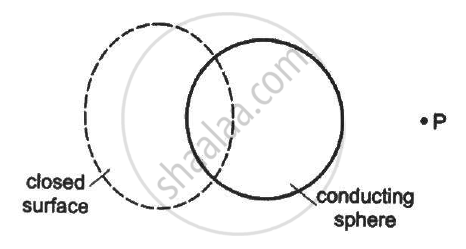Department of Pre-University Education, Karnataka course PUC Karnataka Science Class 12
Share

# The Folloeing Figure Shows a Closed Surface that Intersects a Conducting Sphere. If a Positive Charge is Placed at Point P, - Physics

ConceptElectric Flux

#### Question

The following figure shows a closed surface that intersects a conducting sphere. If a positive charge is placed at point P, the flux of the electric field through the closed surface•  will remain zero

• will become positive

•  will become negative

• will become undefined

#### Solution

will become positive

A positive charge at point P will induce a negative charge on the near face of the conducting sphere, whereas the positive charge on the farther end of the sphere. As this father end is enclosed or intersected by the closed surface, so the flux through it will become positive due to the induced positive charge.

Is there an error in this question or solution?

#### APPEARS IN

HC Verma Solution for Concepts of Physics - Vol. 2 (2018 to Current)
Chapter 8: Gauss’s Law
MCQ | Q: 8 | Page no. 141

#### Video TutorialsVIEW ALL 

Solution The Folloeing Figure Shows a Closed Surface that Intersects a Conducting Sphere. If a Positive Charge is Placed at Point P, Concept: Electric Flux.
S